• PolyFit重建管道 PolyFit实现了以下所述的基于假设和选择的曲面重建方法： Liangliang Nan and Peter Wonka. PolyFit: Polygonal Surface Reconstruction from Point Clouds. ICCV 2017. 如果使用代码/程序（或...
• POLYFIT3（X，Y，N，NUL，W）在最小二乘意义上找到适合数据的度N的多项式P（X）的系数P（X（I））〜= Y（I） . 可以将任何系数强制为零，并且可以对数据进行加权。 NUL 是一个向量，其系数按通常顺序强制为零。 W ...matlab
• 主要介绍了python多项式拟合之np.polyfit 和 np.polyld的实例代码，python数据拟合主要可采用numpy库，库的安装可直接用pip install numpy等,需要的朋友跟随小编一起学习吧
• Polyfit0具有与polyfit相同的功能，不同之处在于拟合的多项式没有常数项，因此可以通过零。 这在身体上通常是正确的。matlab
• 多次拟合任意函数曲线，采用高斯曲线拟合算法求解拟合系数。
• matlab自带函数polyfit的改进版本，采用正交基求解法方程，因而没有病态误差
• POLYFIT_ROOTS 最小二乘多项式拟合数据。 [R，K] = POLYFIT_ROOTS（X，Y，N，TOL）求根R和常数K，因此多项式P（s）= K *（sR（1））*（sR（2））* .. . *(sR(N)) 是数据 Y 在 X 点的最佳最小二乘拟合。 参数 TOL ...matlab
• 功能与 GRABIT 相同，但添加了一项功能以在图像和数据点上绘制 polyfit。 如果不知道拟合的顺序，并且您希望查看原始图像上的拟合，这将很有用。 原始GRABIT指示如下： 应采取以下步骤： 1. 加载图像文件。 2. ...matlab
• 我们提出了一种新的框架，用于从点云重建轻质多边形表面。与传统的方法不同，这些方法既可以提取好的几何原语，也可以获得对原语的适当的排列，而这种方法的重点在于将原语（仅是平面）交叉，并寻找合适的组合，以...重构
• POLYPARCI 使用“polyfit”文档中概述的程序来计算协方差矩阵，并使用“betainc”和“fzero”来计算给定概率和自由度的累积 t 分布和逆 t 分布。 它仅使用核心 MATLAB 函数，不需要统计工具箱。 与统计工具箱函数“t...matlab
• 使用 polyFit2D.m 将二维多项式拟合到数据并使用 polyVal2D.m 对其进行评估。 乐趣！ 此处详细解释了这些功能： http://poquitopicante.blogspot.com/2013/03/horners-method-in-2d.html 它们也托管在 Github 上...matlab
• polyfit.js 用于node.js的多项式拟合求解器库 受到Paul Lutus（ ）的极大启发 : 这只是对这个库进行节点化/组件化并对其格式进行一些改进的一种方法。 用法 要获得可以传递x值并获得相应y值的多项式函数（参数为...JavaScript
• 数学建模--多项式拟合（python实现） 代码中对于给定数据进行不同次数的多项式拟合，并比较了高次多项式拟合可能出现的一些问题。
• matlab开发-polyfit3。将多项式拟合到数据中，使新特征强制为零系数和数据权重。
• python2 / 3：无需任何第三方库（例如numpy，scipy等）即可计算polyfit（1D，2D，ND），也可用于最小二乘解计算和A = QR矩阵分解。 经过python 2.7和3.4的测试，考虑捐赠给该项目：...开源软件
• 用C语言实现polyfit多项式拟合,已知离散点上的数据集，即已知在点集上的函数值，构造一个解析函数（其图形为一曲线）使在原离散点上尽可能接近给定的值。
• 拟合方法——np.polyfit、np.poly1d     今天来介绍一下np.polyfit、np.poly1d 1. np.polyfit  1. 概念 np.polyfit使用的是最小二乘法进行多项式拟合 先看看np.polyfit的参数: numpy....
拟合方法——np.polyfit、np.poly1d
今天来介绍一下np.polyfit、np.poly1d
1. np.polyfit
1. 概念
np.polyfit使用的是最小二乘法进行多项式拟合 先看看np.polyfit的参数:
numpy.polyfit（x，y，deg，rcond = None，full = False，w = None，cov = False ）

参数:
x: 类数组，形状（M，），表示M个采样点的x坐标
y: 类似array_，形状为（M，）或（M，K）, 表示采样点的y坐标。通过传递每列包含一个数据集的2D数组，可以一次拟合多个共享相同x坐标的采样点数据集
deg: 度：整数, 表示拟合多项式的度
cov: cov：布尔值，可选。返回估算值和估算值的协方差矩阵。如果full为True，则不返回cov

返回值:
p: ndarray，形状（deg + 1，）或（deg + 1，K）。项式系数，最高幂优先。

简单介绍几个常用的参数，其他的参数如果想要了解，可以去官网看看: np.polyfit官网
2. 使用
x = np.array([ 0.0 ， 1.0 ， 2.0 ， 3.0 ，  4.0 ，  5.0 ])
y = np.array([ 0.0 ， 0.8 ， 0.9 ， 0.1 ， - 0.8 ， - 1.0 ])
z = np.polyfit(x, y, 3)
print(z)

结果:
array([0.08703704, -0.81349206, 1.69312169, -0.03968254])

即: 0.08703704为最高次

x

3

x^3

的系数，-0.81349206为

x

2

x^2

的系数，1.69312169为

x

x

的系数，-0.03968254为常数项
2. np.poly1d
1. 概念
np.poly1d的参数:
numpy.poly1d（c_or_r，r = False，变量= None ）

参数:
c_or_r: 数组array，代表多项式的系数，以幂次递减，或者如果第二个参数的值为True，则为多项式的根（多项式求值为0的值）。
r bool(可选): 如果为True，则c_or_r指定多项式的根。默认值为False。
可变str(可选): 将打印p时使用的变量从x更改为variable 。

返回值: 相应的多项式

2. 使用
构造多项式

x

2

+

2

x

+

3

x^2+2x+3

coff = np.array([1, 2, 3])
p = np.pyly1d(coff)
print(p)

结果为:
2
1 X + 2 X + 3
 给定x的值，求出多项式的值(比如给出x=0.5)print(p(0.5))

结果为:
4.5
 显示多项式系数print(p.c)

结果为:
array([1, 2, 3])
 显示多项式中的第k次幂的系数print(p)
print(p)
print(p)

结果为:
1
2
3
 多项式可以相加，相减，相乘和相除(返回商和余数):print(p * p)
print(p**2)
print(np.square(p))

结果为:
poly1d([ 1,  4, 10, 12,  9])
poly1d([ 1,  4, 10, 12,  9])
array([1, 4, 9])
 可以使用参数variable修改p的字符串表示形式中使用的变量：c= np.poly1d([1,2,3], variable='z')
print(c)

结果为:
2
1 z + 2 x + 3
 当第二个参数r为True时, 传入的数组中的数为多项式的根:print(poly1d([1, 2, 3], True))

结果为:
3     2
1 x - 6 x + 11 x - 6


x

3

−

6

x

2

+

11

x

−

6

x^3-6x^2+11x-6

=

(

x

−

1

)

(

x

−

2

)

(

x

−

3

)

(x-1)(x-2)(x-3)

3. 使用np.polyfit、np.poly1d进行拟合
先说明一下，以下代码中使用的拟合优度计算方法以及代码在我的上一篇博客中可以找到: 数学建模方法—【03】拟合优度的计算(python计算) 使用np.polyfit、np.poly1d进行1-5阶的拟合:
拟合数据集
x = np.array([4, 8, 10, 12, 25, 32, 43, 58, 63, 69, 79])
y = np.array([20, 33, 50, 56, 42, 31, 33, 46, 65, 75, 78])

一阶拟合:
figure1 = plt.figure(figsize=(8, 6))
# coeff 为系数，poly_fit 拟合函数
# 1. 先拟合获取系数
coeff_1 = np.polyfit(x, y, 1)
print("一阶拟合系数为:", coeff_1)
# 2. 根据系数得到多项式
poly_fit_1 = np.poly1d(coeff_1)
print("一阶多项式为:", poly_fit_1)
# 3. 输入变量(单个值或者变量数组)，得到拟合结果(数组)
y_fit_1 = poly_fit_1(x)
print("拟合得到的数据为L", y_fit_1)
# 4. 根据结果作图
plt.plot(x, y_fit_1, 'green',label="一阶拟合")
# 5. 根据原始数据以及拟合数据得到拟合优度
rr1 = goodness_of_fit(y_fit_1, y)
print("拟合优度为%.5f" % rr1)

输出结果为:
一阶拟合系数为: [ 0.5000123  29.77227674]
一阶多项式为:
0.5 x + 29.77
一阶拟合得到的数据为:  [31.77232593 33.77237512 34.77239971 35.77242431 42.27258418 45.77267026
51.27280553 58.77298999 61.27305148 64.27312526 69.27324824]
一阶拟合优度为0.52079

二阶拟合:
## 二阶拟合
coeff_2 = np.polyfit(x, y, 2)
print("二阶拟合系数为:", coeff_2)
poly_fit_2 = np.poly1d(coeff_2)
print("二阶多项式为:", poly_fit_2)
y_fit_2 = poly_fit_2(x)
print("二阶拟合得到的数据为: ", y_fit_2)
plt.plot(x, y_fit_2, 'orange',label="二阶拟合")
rr2 = goodness_of_fit(y_fit_2, y)
print("拟合优度为%.5f" % rr2)

结果为:
二阶拟合系数为: [ 1.31325973e-02 -5.41278736e-01  4.14687611e+01]
二阶多项式为:          2
0.01313 x - 0.5413 x + 41.47
二阶拟合得到的数据为:  [39.51376772 37.97901745 37.36923348 36.86451029 36.14466602 37.59562119
42.47594787 54.25265174 59.49147943 66.64482408 80.66828073]
二阶拟合优度为0.64095

三阶拟合:
## 三阶拟合
coeff_3 = np.polyfit(x, y, 3)
print("三阶拟合系数为:", coeff_3)
poly_fit_3 = np.poly1d(coeff_3)
print("三阶多项式为:", poly_fit_3)
y_fit_3 = poly_fit_3(x)
print("三阶拟合得到的数据为: ", y_fit_3)
plt.plot(x, y_fit_3, 'skyblue',label="三阶拟合")
rr3 = goodness_of_fit(y_fit_3, y)
print("拟合优度为%.5f" % rr3)

结果为:
三阶拟合系数为: [ 3.52702455e-04 -3.04653592e-02  8.94352257e-01  3.18682147e+01]
三阶多项式为:            3           2
0.0003527 x - 0.03047 x + 0.8944 x + 31.87
三阶拟合得到的数据为:  [34.98075096 37.25383345 38.11790383 38.82289993 40.69714753 40.84831321
42.03722677 50.07165879 55.48758714 64.39887624 86.28380214]
三阶拟合优度为0.67675

四阶拟合:
## 四阶拟合
coeff_4 = np.polyfit(x, y, 4)
print("四阶拟合系数为:", coeff_4)
poly_fit_4 = np.poly1d(coeff_4)
print("四阶多项式为:", poly_fit_4)
y_fit_4 = poly_fit_4(x)
print("四阶拟合得到的数据为: ", y_fit_4)
plt.plot(x, y_fit_4, 'blue',label="四阶拟合")
rr4 = goodness_of_fit(y_fit_4, y)
print("拟合优度为%5f" % rr4)

结果为:
四阶拟合系数为: [-5.20382218e-05  9.18774157e-03 -5.17764921e-01  1.03856517e+01
-1.43837752e+01]
四阶多项式为:             4            3          2
-5.204e-05 x + 0.009188 x - 0.5178 x + 10.39 x - 14.38
四阶拟合得到的数据为:  [19.44928636 40.05545822 46.36360859 50.48324889 44.88547204 34.26368367
27.43335073 49.97112982 62.51444419 75.84652392 77.73379358]
四阶拟合优度为0.953101

五阶拟合:
## 五阶拟合
coeff_5 = np.polyfit(x, y, 5)
print("五阶拟合系数为:", coeff_5)
poly_fit_5 = np.poly1d(coeff_5)
print("五阶多项式为:", poly_fit_5)
y_fit_5 = poly_fit_5(x)
print("五阶拟合得到的数据为: ", y_fit_5)
plt.plot(x, y_fit_5, 'red', label="五阶拟合")
rr5 = goodness_of_fit(y_fit_5, y)
print("拟合优度为%.5f" % rr5)

结果为:
五阶拟合系数为: [ 2.92948996e-07 -1.11412559e-04  1.34633715e-02 -6.48604067e-01
1.19810057e+01 -2.01221591e+01]
五阶多项式为:            5             4           3          2
2.929e-07 x - 0.0001114 x + 0.01346 x - 0.6486 x + 11.98 x - 20.12
五阶拟合得到的数据为:  [18.25763258 40.66172555 47.20603154 51.2782733  43.7309192  33.27241619
28.39304966 51.21670562 62.50290083 74.18143391 78.29891163]
五阶拟合优度为0.95620

作图：
plt.scatter(x, y, color='black', label="原始数据")
plt.title("1~5阶拟合曲线图")
plt.rcParams['font.sans-serif'] = ['SimHei']  # 用来正常显示中文标签
plt.rcParams['axes.unicode_minus'] = False  # 用来正常显示负号
plt.legend(loc=2)
plt.show()

结果为:所有代码:
# 拟合数据集
x = np.array([4, 8, 10, 12, 25, 32, 43, 58, 63, 69, 79])
y = np.array([20, 33, 50, 56, 42, 31, 33, 46, 65, 75, 78])

figure1 = plt.figure(figsize=(8, 6))
# coeff 为系数，poly_fit 拟合函数
# 1. 先拟合获取系数
coeff_1 = np.polyfit(x_arr, y_arr, 1)
print("一阶拟合系数为:", coeff_1)
# 2. 根据系数得到多项式
poly_fit_1 = np.poly1d(coeff_1)
print("一阶多项式为:", poly_fit_1)
# 3. 输入变量(单个值或者变量数组)，得到拟合结果(数组)
y_fit_1 = poly_fit_1(x_arr)
print("一阶拟合得到的数据为: ", y_fit_1)
# 4. 根据结果作图
plt.plot(x_arr, y_fit_1, 'green',label="一阶拟合")
# 5. 根据原始数据以及拟合数据得到拟合优度
rr1 = goodness_of_fit(y_fit_1, y_arr)
print("一阶拟合优度为%.5f" % rr1)
#
coeff_2 = np.polyfit(x_arr, y_arr, 2)
print("二阶拟合系数为:", coeff_2)
poly_fit_2 = np.poly1d(coeff_2)
print("二阶多项式为:", poly_fit_2)
y_fit_2 = poly_fit_2(x_arr)
print("二阶拟合得到的数据为: ", y_fit_2)
plt.plot(x_arr, y_fit_2, 'orange',label="二阶拟合")
rr2 = goodness_of_fit(y_fit_2, y_arr)
print("二阶拟合优度为%.5f" % rr2)
# #
coeff_3 = np.polyfit(x_arr, y_arr, 3)
print("三阶拟合系数为:", coeff_3)
poly_fit_3 = np.poly1d(coeff_3)
print("三阶多项式为:", poly_fit_3)
y_fit_3 = poly_fit_3(x_arr)
print("三阶拟合得到的数据为: ", y_fit_3)
plt.plot(x_arr, poly_fit_3(x_arr), 'skyblue',label="三阶拟合")
rr3 = goodness_of_fit(y_fit_3, y_arr)
print("三阶拟合优度为%.5f" % rr3)
# #
coeff_4 = np.polyfit(x_arr, y_arr, 4)
print("四阶拟合系数为:", coeff_4)
poly_fit_4 = np.poly1d(coeff_4)
print("四阶多项式为:", poly_fit_4)
y_fit_4 = poly_fit_4(x_arr)
print("四阶拟合得到的数据为: ", y_fit_4)
plt.plot(x, y_fit_4, 'blue',label="四阶拟合")
rr4 = goodness_of_fit(y_fit_4, y_arr)
print("四阶拟合优度为%5f" % rr4)
#
coeff_5 = np.polyfit(x_arr, y_arr, 5)
print("五阶拟合系数为:", coeff_5)
poly_fit_5 = np.poly1d(coeff_5)
print("五阶多项式为:", poly_fit_5)
y_fit_5 = poly_fit_5(x_arr)
print("五阶拟合得到的数据为: ", y_fit_5)
plt.plot(x_arr, y_fit_5, 'red', label="五阶拟合")
rr5 = goodness_of_fit(y_fit_5, y_arr)
print("五阶拟合优度为%.5f" % rr5)

plt.scatter(x, y, color='black', label="原始数据")
plt.title("1~5阶拟合曲线图")
plt.rcParams['font.sans-serif'] = ['SimHei']  # 用来正常显示中文标签
plt.rcParams['axes.unicode_minus'] = False  # 用来正常显示负号
plt.legend(loc=2)
plt.show()

展开全文python 数学建模 数据分析 多项式拟合
• MATLAB工具箱中提供了最小二乘拟合函数 polyfit() -->多项式曲线拟合 具体调用格式有三种： P = polyfit(X,Y,N) [P,S] = polyfit(X,Y,N) [P,S,MU] = polyfit(X,Y,N) （1）P = polyfit(X,Y,N) 返回次数为 n 的多项式...
解决数据拟合问题最重要方法是最小二乘法和回归分析。如，我们需要从一组测定的数据（例如N个点（xi，yi）（i=0,1，…，m））去求得自变量 x 和因变量 y 的一个近似解表达式 y=f（x），这就是由给定的 N 个点（xi，yi）（i=0,1，…，m）求数据拟合的问题。（注意数据拟合和数据插值是不同的，举个例子：因为测量数据往往不可避免地带有测试误差，而插值多项式又通过所有的点（xi，yi），这样就使插值多项式保留了这些误差，从而影响逼近精度，使得插值效果不理想） 所以使用最小二乘法曲线拟合法：即寻求已知函数的一个逼近函数y=f（x），使得逼近函数从总体与已知函数的偏差按某种方法度量能达到最小，而又不一定通过全部的点（xi，yi），这个时候就需要使用最小二乘法曲线拟合法。 数据拟合的具体做法是：对给定的数据（xi，yi）（i=0,1，…，m），在取定的函数类

ϕ

\phi

中使误差

r

i

=

p

(

x

i

)

−

y

i

(

i

=

0

,

1

,

…

,

m

)

r_{i}=p\left(x_{i}\right)-y_{i}(i=0,1, \ldots, m)

的平方和最小，即

[

∑

i

=

0

m

r

i

2

=

∑

i

=

0

m

[

p

(

x

i

−

y

i

)

]

2

]

min

⁡

\left[\sum_{i=0}^{m} r_{i}^{2}=\sum_{i=0}^{m}\left[p\left(x_{i}-y_{i}\right)\right]^{2}\right]_{\min }

从几何意义讲，即寻求与给定点

x

i

−

y

i

(

i

=

0

,

1

,

…

,

m

)

x_{i}-y_{i}(i=0,1, \ldots, m)

的距离平方和为最小的曲线y=p（x）。函数p（x）称为拟合函数或最小二乘解，求拟合函数p（x）的方法称为曲线拟合的最小二乘法。 在曲线拟合中，函数类

ϕ

\phi

可有不同的选取方法。 MATLAB工具箱中提供了最小二乘拟合函数 polyfit() -->多项式曲线拟合 具体调用格式有三种：
P = polyfit(X,Y,N)[P,S] = polyfit(X,Y,N)[P,S,MU] = polyfit(X,Y,N)

（1）P = polyfit(X,Y,N) 返回次数为 n 的多项式 p(x) 的系数，该阶数是 y 中数据的最佳拟合（在最小二乘方式中）。p 中的系数按降幂排列，p 的长度为 n+1. 其中 X 为输入的向量x，Y 为得到的函数值，N 表示拟合的最高次数，返回的P值为拟合的多项式：

P

(

1

)

×

X

n

+

P

(

2

)

×

X

n

−

1

+

…

+

P

(

N

)

×

X

+

P

(

N

+

1

)

P(1) \times X^{n}+P(2) \times X^{n-1}+\ldots+P(N) \times X+P(N+1)

（2） [P,S] = polyfit(X,Y,N)还返回一个结构体 S，S可用作 polyval 的输入来获取误差估计值（S为由范德蒙矩阵的QR分解的R分量）。 其中 X 为输入的向量x，Y 为得到的函数值，N 表示拟合的最高次数，返回的P值为拟合的多项式：

P

(

1

)

×

X

n

+

P

(

2

)

×

X

n

−

1

+

…

+

P

(

N

)

×

X

+

P

(

N

+

1

)

P(1) \times X^{n}+P(2) \times X^{n-1}+\ldots+P(N) \times X+P(N+1)

（3）[P,S,MU] = polyfit(X,Y,N)还返回 mu，mu是一个二元素向量，包含中心化值和缩放值。mu(1) 是 mean(x)，mu(2) 是 std(x)。使用这些值时，polyfit 将 x 的中心置于零值处并缩放为具有单位标准差：

x

^

=

x

−

x

ˉ

σ

x

\hat{x}=\frac{x-\bar{x}}{\sigma_{x}}

这种中心化和缩放变换可同时改善多项式和拟合算法的数值属性。

下面来看一些具体的例子：（来源于帮助文档，改编）
将多项式与三角函数拟合： 在区间 [0,4*pi] 中沿余弦曲线生成 10 个等间距的点。
>> x = linspace(0,4*pi,10);
>> y = cos(x);

使用 polyfit 将一个 7 次多项式与这些点拟合。
>> p = polyfit(x,y,7);

在更精细的网格上计算多项式并绘制结果图。
>> x1 = linspace(0,4*pi);
>> y1 = polyval(p,x1);
>> figure
>> plot(x,y,'o')
>> hold on
>> plot(x1,y1,'m')
>> hold off

运行结果如下：将多项式与点集拟合 创建一个由区间 [0,1] 中的 5 个等间距点组成的向量，并计算这些点处的 y（x）= (2+x)^-1 。
>> x=0:0.2:1;   //或者写成  >> x=0:0.2:1;  也可以实现相同的作用
>> y = 1./(2+x);

将 4 次多项式与 5 个点拟合。通常，对于 n 个点，可以拟合 n-1 次多项式以便完全通过这些点。
>> p = polyfit(x,y,4);

在由 0 和 2 之间的点组成的更精细网格上计算原始函数和多项式拟合。
>> x1 = linspace(0,2);
>> y1 = 1./(2+x1);
>> f1 = polyval(p,x1);

在更大的区间 [0,2] 中绘制函数值和多项式拟合，其中包含用于获取以圆形突出显示的多项式拟合的点。多项式拟合在原始 [0,1] 区间中的效果较好，但在该区间外部很快与拟合函数出现差异。
>> figure
>> plot(x,y,'o')
>> hold on
>> plot(x1,y1)
>> plot(x1,f1,'b--')
>> legend('y','y1','f1')

运行结果如下：对误差函数进行多项式拟合： 首先生成 x 点的向量，在区间 [0,1.5*pi] 内等间距分布；然后计算这些点处的 erf(x)。 注：erf 是误差函数，也称高斯误差函数。
x = (0:0.10:1.5*pi)';
y=erf(x);

确定6次逼近多项式的系数。
p=polyfit(x,y,6)

p =
0.0019   -0.0259    0.1242   -0.1785   -0.3442    1.2684   -0.0095

为了查看拟合情况如何，在各数据点处计算多项式，并生成说明数据、拟合和误差的一个表。
f=polyval(p,x);
T=table(x,y,f,y-f,'VariableNames',{'X','Y','Fit','FitError'})

T =
48×4 table
 X        Y          Fit         FitError
___    _______    __________    ___________

0          0    -0.0094811      0.0094811
0.1    0.11246       0.11375     -0.0012876
0.2     0.2227       0.22919     -0.0064911
0.3    0.32863       0.33619     -0.0075599
0.4    0.42839       0.43431      -0.005914
0.5     0.5205       0.52334     -0.0028408
0.6    0.60386       0.60326     0.00059392
0.7     0.6778        0.6742      0.0035981
0.8     0.7421       0.73643      0.0056695
0.9    0.79691       0.79033      0.0065794
1     0.8427       0.83637      0.0063316
1.1    0.88021        0.8751      0.0051058
1.2    0.91031       0.90712       0.003194
1.3    0.93401       0.93307     0.00093826
1.4    0.95229       0.95361      -0.001323
1.5    0.96611        0.9694     -0.0032971
...

在该区间中，插值与实际值非常符合。创建一个绘图，以显示在该区间以外，外插值与实际数据值如何快速偏离。
 x1=(0:0.10:2*pi)';
y1=erf(x1);
f1=polyval(p,x1);
plot(x,y,'o')
plot(x1,y1,'-')
hold on
plot(x1,y1,'-')
plot(x1,f1,'g-')
axis([0  5.5  0  3])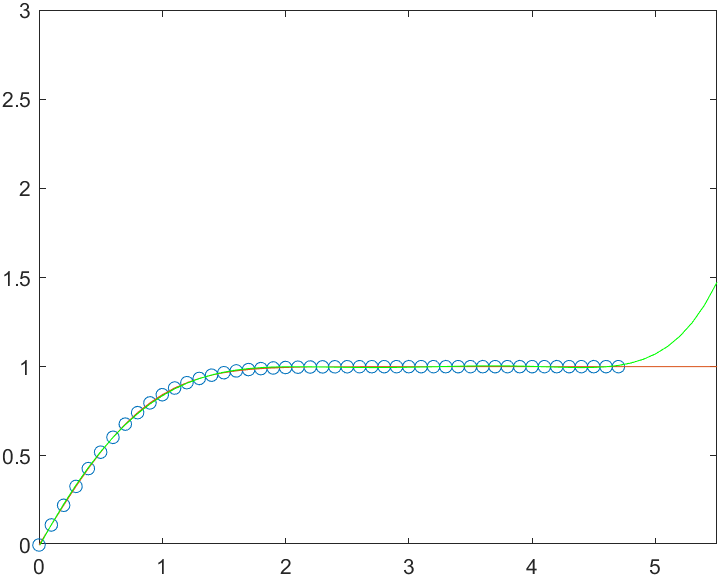使用中心化和缩放改善数值属性 创建一个由 1750 - 2000 年的人口数据组成的表，并绘制数据点。
 year = (1750:25:2000)';
pop = 1e6*[791 856 978 1050 1262 1544 1650 2532 6122 8170 11560]';
T = table(year, pop)

T =
11×2 table
year       pop
____    _________

1750     7.91e+08
1775     8.56e+08
1800     9.78e+08
1825     1.05e+09
1850    1.262e+09
1875    1.544e+09
1900     1.65e+09
1925    2.532e+09
1950    6.122e+09
1975     8.17e+09
2000    1.156e+10

 plot(year,pop,'square')使用带三个输入的 polyfit 拟合一个使用中心化和缩放的 5 次多项式，这将改善问题的数值属性。polyfit 将 year 中的数据以 0 为进行中心化，并缩放为具有标准差 1，这可避免在拟合计算中出现病态的 Vandermonde （范德蒙）矩阵。
[p,~,mu] = polyfit(T.year, T.pop, 5);

使用带四个输入的 polyval，根据缩放后的年份 (year-mu(1))/mu(2) 计算 p。绘制结果对原始年份的图。
f = polyval(p,year,[],mu);
hold on
plot(year,f)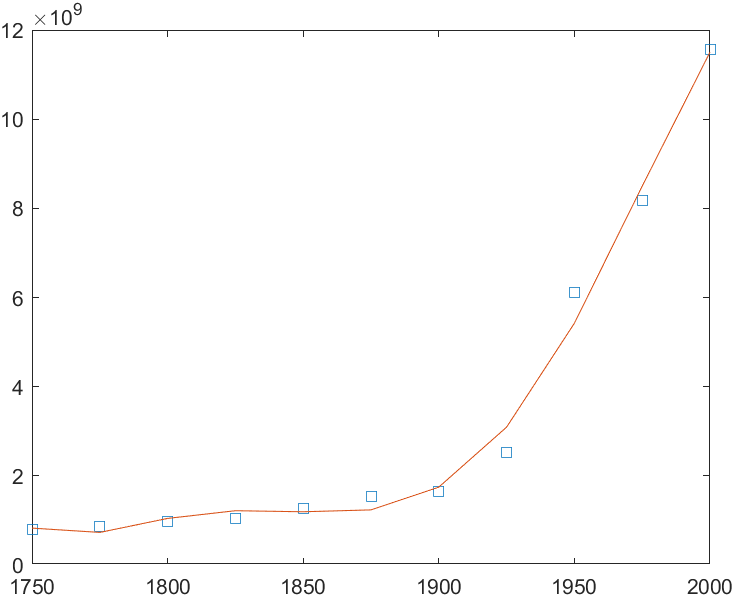简单线性回归 将一个简单线性回归模型与一组离散二维数据点拟合。 创建几个由样本数据点 (x,y) 组成的向量。对数据进行一次多项式拟合。
>> x=1:40;
>> y=0.4*x-1.5*randn(1,60);
注意：这里 矩阵维度必须一致。 所以第二行代码的输入是错误的

y=0.4*x-1.5*randn(1,40);
p=polyfit(x,y,1);

计算在 x 中的点处拟合的多项式 p。用这些数据绘制得到的线性回归模型。
 f = polyval(p,x);
plot(x,y,'o',x,f,'-')
legend('data','linear fit')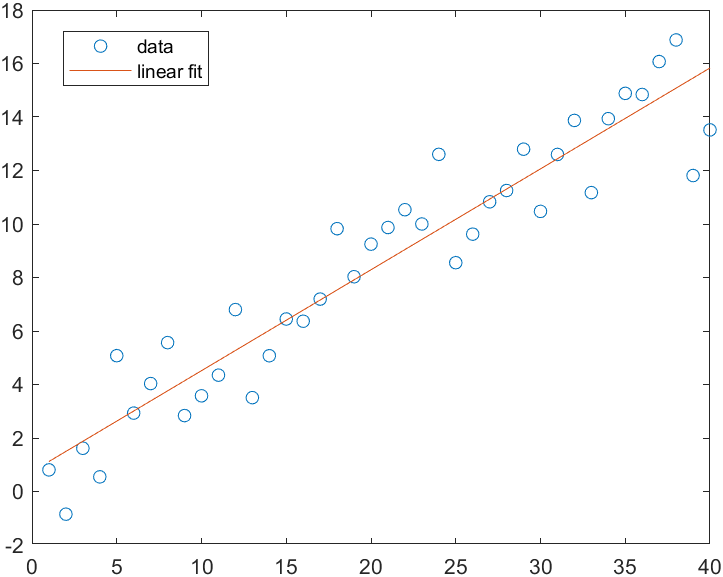具有误差估计值的线性回归 将一个线性模型拟合到一组数据点并绘制结果，其中包含预测区间为 95% 的估计值。 创建几个由样本数据点 (x,y) 组成的向量。使用 polyfit 对数据进行一次多项式拟合。指定两个输出以返回线性拟合的系数以及误差估计结构体。
x = 1:100;
y = -0.3*x + 2*randn(1,100);
[p,S] = polyfit(x,y,1);

计算以 p 为系数的一次多项式在 x 中各点处的拟合值。将误差估计结构体指定为第三个输入，以便 polyval 计算标准误差的估计值。标准误差估计值在 delta 中返回。
[y_fit,delta] = polyval(p,x,S);

绘制原始数据、线性拟合和 95% 预测区间

y

±

2

Δ

y \pm 2 \Delta

。
plot(x,y,'go')
hold on
plot(x,y_fit,'r-')
plot(x,y_fit+2*delta,'m--',x,y_fit-2*delta,'m--')
title('Linear Fit of Data with 95% Prediction Interval')
legend('Data','Linear Fit','95% Prediction Interval')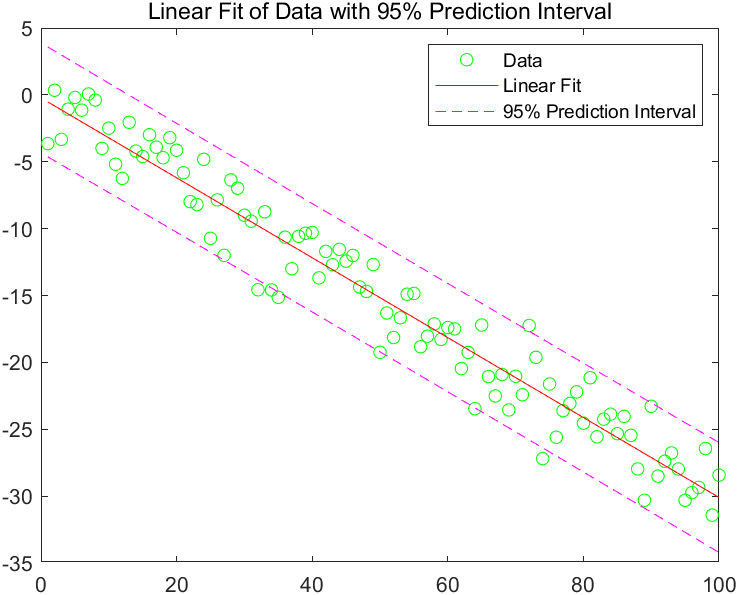输入参数解释： x – 查询点 （向量） 查询点，指定为一个向量。x 中的点对应于 y 中包含的拟合函数值。如果 x 不是向量，则 polyfit 将其转换为列向量 x( : )。😃 x 具有重复（或接近重复）的点或者如果 x 可能需要中心化和缩放时的警告消息结果。 **数据类型：**single | double 复数支持： 是 y – 查询点位置的拟合值 （向量） 查询点位置的拟合值，指定为向量。y 中的值对应于 x 中包含的查询点。如果 y 不是向量，则 polyfit 将其转换为列向量 y ( : ) 。😃 **数据类型：**single | double 复数支持： 是 n --多项式拟合的次数 （正整数标量） 多项式拟合的次数，指定为正整数标量。n 指定 p 中最左侧系数的多项式幂。 输出参数解释： p – 最小二乘拟合多项式系数（向量）
最小二乘拟合多项式系数，以向量的形式返回。p 的长度为 n+1，包含按降幂排列的多项式系数，最高幂为 n。如果 x 或 y 包含 NaN 值且 n < length(x)，则 p 的所有元素均为 NaN。
使用 polyval 计算 p 在查询点处的解。
S – 误差估计结构体 （结构体） 误差估计结构体。此可选输出结构体主要用作 polyval 函数的输入，以获取误差估计值。S 包含以下字段：
字段说明RVandermonde 矩阵 x 的 QR 分解的三角因子df自由度normr残差的范数
如果 y 中的数据是随机的，则 p 的估计协方差矩阵是 (Rinv*Rinv’)*normr^2/df，其中 Rinv 是 R 的逆矩阵。
如果 y 中数据的误差呈独立正态分布，并具有常量方差，则 [y,delta] = polyval(…) 可生成至少包含 50% 的预测值的误差边界。即 y ± delta 至少包含 50% 对 x 处的未来观测值的预测值。
mu – 中心化值和缩放值
中心化值和缩放值，以二元素向量形式返回。mu(1) 为 mean(x)，mu(2) 为 std(x)。这些值以单位标准差将 x 中的查询点的中心置于零值处。
使用 mu 作为 polyval 的第四个输入以计算 p 在缩放点 (x - mu(1))/mu(2) 处的解。
局限性
在涉及很多点的问题中，使用 polyfit 增加多项式拟合的次数并不总能得到较好的拟合。高次多项式可以在数据点之间振动，导致与数据之间的拟合较差。在这些情况下，可使用低次多项式拟合（点之间倾向于更平滑）或不同的方法，具体取决于该问题。多项式在本质上是无边界的振荡函数。所以，它们并不非常适合外插有界的数据或单调（递增或递减）的数据。
算法： polyfit 使用 x 构造具有 n+1 列和 m = length(x) 行的 Vandermonde 矩阵 V 并生成线性方程组

(

x

1

n

x

1

n

−

1

…

1

n

2

x

2

−

1

…

1

⋮

⋮

⋱

⋮

n

m

x

m

−

1

…

1

)

(

p

1

p

2

⋮

p

n

+

1

)

=

(

y

1

y

2

⋮

y

m

)

\left(\begin{array}{cccc} x_{1}^{n} & x_{1}^{n-1} & \ldots & 1 \\ n_{2} & x_{2}-1 & \ldots & 1 \\ \vdots & \vdots & \ddots & \vdots \\ n_{m} & x_{m}-1 & \ldots & 1 \end{array}\right)\left(\begin{array}{c} p_{1} \\ p_{2} \\ \vdots \\ p_{n+1} \end{array}\right)=\left(\begin{array}{c} y_{1} \\ y_{2} \\ \vdots \\ y_{m} \end{array}\right)

其中 polyfit 使用 p = V\y 求解。由于 Vandermonde 矩阵中的列是向量 x 的幂，因此条件数 V 对于高阶拟合来说通常较大，生成一个奇异系数矩阵。在这些情况下，中心化和缩放可改善系统的数值属性以产生更可靠的拟合。
展开全文matlab 编程语言
• polyfit函数的调用语法是： [p,s]=polyfit(x,y,m) 输入参数x，y为测量而得的原始数据，为向量；m为欲拟合的多项式的次数。polyfit (x,y,m)将根据原始数据x、y得到一个m次拟合多项式P(x)的系数，该多项式能在最小...


4.6  曲线拟合
在上一节，已经介绍了数据插值，它要求原始数据是精确的，或具有较小的误差。事实上，由于种种原因，实验或测量中所获得的数据总会有一定的误差。在这种情况下，如果强求构造的函数(曲线)通过各插值节点，显然是不合理的。为此，人们设想构造一个函数(曲线)y=g(x)去拟合f(x)，但它不必通过各插值节点，而只是使该曲线从这些插值节点中穿过，且使它在某种意义下最优。
MATLAB的曲线拟合是用常见的最小二乘原理，所构造的g(x)是一个次数小于拟合节点个数的多项式。
4.6.1  最小二乘原理及其曲线拟合算法
设测得离散的n+1个节点的数据如下：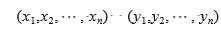构造一个如下的m次拟合多项式函数g(x)为 (m≤n)：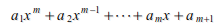所谓曲线拟合的最小二乘原理，就是使上述拟合多项式在各数据点处的偏差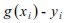的平方之和达最小。
上式中的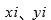均为已知值，而式中的系数为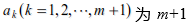个未知数，故可以将其看做是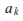的函数，即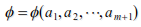。于是我们可以把上述曲线拟合归结成对多元函数的求极值问题。为使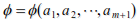取极小值，必须满足以下方程组：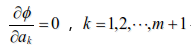经过简单的推导，可以得到一个m+1阶线性代数方程组Sa=t，其中S为m+1阶系数矩阵，t为右端项，而a为未知数向量，即欲求的m次拟合多项式的m+1个系数。这个方程组也称为正则方程组。至于正则方程组的具体推导，可参阅有关数值计算方法的教材。
4.6.2  曲线拟合的实现
在MATLAB中，可以用polyfit函数来求最小二乘拟合多项式的系数，另外可以用polyval函数按所得的多项式计算指定值。
polyfit函数的调用语法是：
[p,s]=polyfit(x,y,m)
输入参数x，y为测量而得的原始数据，为向量；m为欲拟合的多项式的次数。polyfit (x,y,m)将根据原始数据x、y得到一个m次拟合多项式P(x)的系数，该多项式能在最小二乘意义下最优地近似函数f(x)，即有p(xi)≈f(xi)≈yi。
返回的结果中p为m次拟合多项式的系数，而s中的数据则是一个结构数组，代入polyval函数后可以得到拟合多项式相关的误差估计。s最常用的写法可以是：p=polyfit(x,y,M)。
polyval的函数功能是按多项式的系数计算指定点所对应的函数值。
【例4-43】  曲线拟合示例。
本例首先在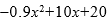多项式的基础上加入随机噪声，产生测试数据，然后对测试数据进行数据曲线拟合：
>> clear
>> rand('state',0)
>> x=1:1:10;
>> y=-0.9*x.^2+10*x+20+rand(1,10).*5; %  产生测试数据
>> plot(x,y,'o')                  %  绘图并标出原始数据点
>> p=polyfit(x,y,2)
>> xi=1:0.5:10;
>> yi=polyval(p,xi);                     % 计算拟合的结果
>> hold on
>> plot(xi,yi);                   %  绘制拟合结果图
>> hold off
运行以上命令，得到的结果如图4-10所示。另外得到的多项式系数为：
p =
-0.8923    9.8067   23.6003
也就是说通过曲线拟合，得到了多项式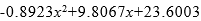。通过比较系数和观察图形，可以看出本次曲线拟合结果的精度是比较高的。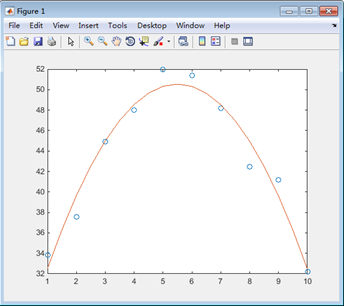图4-10  曲线拟合
4.7  Fourier分析
傅立叶(Fourier)分析在信号处理领域有着广泛的应用，现实生活中大部分的信号都包含有多个不同的频率组件，这些信号组件频率会随着时间或快或慢的变化。傅立叶级数和傅立叶变换是用来分析周期或者非周期信号的频率特性的数学工具。从时间的角度来看，傅立叶分析包括连续时间和离散时间的傅立叶变换，总共有4种不同的傅立叶分析类型：连续时间的傅立叶级数、连续时间的傅立叶变换、离散时间的傅立叶级数、离散时间的傅立叶变换等。
频谱分析是在数据中识别频率组成的处理过程。对于离散数据，频谱分析的计算基础是离散傅立叶变换(DFT)。DFT将time-based或者space-based数据转换为frequency-based数据。
一个长度为n的向量x的DFT，也是一个长度为n的向量：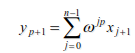其中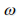是n阶复数根：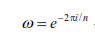在此表达式中，i表示虚数单位 。
DFT有一种快速算法FFT，称为快速傅立叶变换。FFT并不是与DFT不同的另一种变换，而是为了减少DFT运算次数的一种快速算法。它是对变换式进行一次分解，使其成为若干个小数点的组合，从而减少运算量。常用的FFT是以2为基数的，其长度用N表示，N为2的整数倍。
MATLAB中采用的就是FFT算法。MATLAB提供了函数fft和ifft等来进行傅立叶分析。
1．函数fft和ifft
函数fft和ifft对数据作一维快速傅立叶变换和傅立叶反变换，函数fft的调用语法有如下几种。
(1)Y=fft(X)：如果X是向量，则采用快速傅立叶变换算法作X的离散傅立叶变换；如果X是矩阵，则计算矩阵每一列的傅立叶变换。
(2)Y=fft(X,n)：用参数n限制X的长度，如果X的长度小于n，则用0补足；如果X的长度大于n，则去掉长出的部分。
(3)Y=fft(X,[ ],n)或Y=fft(X,n,dim)：在参数dim指定的维上进行操作。
函数ifft的用法和fft完全相同。
2．fft2和ifft2
函数fft2和ifft2对数据作二维快速傅立叶变换和傅立叶反变换。数据的二维傅立叶变换fft2(X)相当于fft(fft(X)’)’，即先对X的列做一维傅立叶变换，然后对变换结果的行做一维傅立叶变换。函数fft2的调用语法有如下几种。
(1)Y=fft2(X)：二维快速傅立叶变换。
(2)Y=fft2(X,MROWS,NCOLS)：通过截断或用0补足，使X成为MROWS*NCOLS的矩阵。
函数ifft2的用法和fft2完全相同。
3．fftshift和ifftshift
函数fftshift(Y)用于把傅立叶变换结果Y(频域数据)中的直流分量(频率为0处的值)移到中间位置：
(1)如果Y是向量，则交换Y的左右半边；
(2)如果Y是矩阵，则交换其一三象限和二四象限；
(3)如果Y是多维数组，则在数组的每一维交换其“半空间”。
函数ifftshift相当于把fftshift函数的操作逆转，用法相同。
【例4-44】  生成一个正弦衰减曲线，进行快速傅立叶变换，并画出幅值(amplitude)图、相位(phase)图、实部(real)图和虚部(image)图。
>> tp=0:2048;                             %  时域数据点数N
>> yt=sin(0.08*pi*tp).*exp(-tp/80);      %  生成正弦衰减函数
>> plot(tp,yt), axis([0,400,-1,1]),      %  绘正弦衰减曲线
>> t=0:800/2048:800;                       %  频域点数Nf
>> f=0:1.25:1000;
>> yf=fft(yt);                             %  快速傅立叶变换
>> ya=abs(yf(1:801));                      %  幅值
>> yp=angle(yf(1:801))*180/pi;            %  相位
>> yr=real(yf(1:801));                    %  实部
>> yi=imag(yf(1:801));                     %  虚部
>> figure
>> subplot(2,2,1)
>> plot(f,ya),axis([0,200,0,60])        %  绘制幅值曲线
>> title('幅值曲线')
>> subplot(2,2,2)
>> plot(f,yp),axis([0,200,-200,10])     %  绘制相位曲线
>> title('相位曲线')
>> subplot(2,2,3)
>> plot(f,yr),axis([0,200,-40,40])      %  绘制实部曲线
>> title('实部曲线')
>> subplot(2,2,4)
>> plot(f,yi),axis([0,200,-60,10])      %  绘制虚部曲线
>> title('虚部曲线')
本例首先生成正弦衰减函数yt，绘制的正弦衰减曲线如图4-11所示。然后对yt进行了快速傅立叶变换，结果如图4-12所示。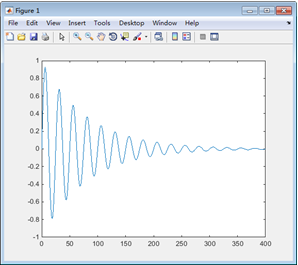图4-11  正弦衰减曲线图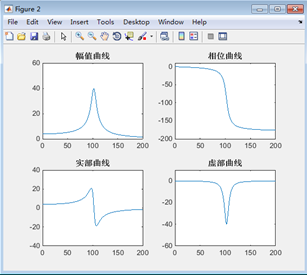图4-12  傅立叶变换结果展开全文matlab复数信号
• from numpy\lib\polynomial...@array_function_dispatch(_polyfit_dispatcher) def polyfit(x, y, deg, rcond=None, full=False, w=None, cov=False): """ Least squares polynomial fit. 最小二乘多项式拟合。 ...
from numpy\lib\polynomial.py
@array_function_dispatch(_polyfit_dispatcher)
def polyfit(x, y, deg, rcond=None, full=False, w=None, cov=False):
"""
Least squares polynomial fit.
最小二乘多项式拟合。

Fit a polynomial p(x) = p * x**deg + ... + p[deg] of degree deg to points (x, y). Returns a vector of coefficients p that minimises the squared error in the order deg, deg-1, ... 0.
将度数deg''的多项式p（x）= p  * x ** deg + ... + p°''拟合到点（x，y）。 返回系数p的向量，该向量按deg，deg-1，... 0的顺序最小化平方误差。

The Polynomial.fit <numpy.polynomial.polynomial.Polynomial.fit> class method is recommended for new code as it is more stable numerically. See the documentation of the method for more information.
对于新代码，建议使用Polynomial.fit <numpy.polynomial.polynomial.Polynomial.fit>类方法，因为它在数值上更稳定。 有关更多信息，请参见该方法的文档。

Parameters
----------
x : array_like, shape (M,)
x-coordinates of the M sample points (x[i], y[i]).
M个采样点的（x [i]，y [i]）''的x坐标。
y : array_like, shape (M,) or (M, K)
y-coordinates of the sample points. Several data sets of sample points sharing the same x-coordinates can be fitted at once by passing in a 2D-array that contains one dataset per column.
采样点的y坐标。 可以通过传入每列包含一个数据集的2D数组，一次拟合几个共享相同x坐标的采样点数据集。
deg : int
Degree of the fitting polynomial
拟合多项式的度（阶数？）
rcond : float, optional
Relative condition number of the fit. Singular values smaller than this relative to the largest singular value will be ignored. The default value is len(x)*eps, where eps is the relative precision of the float type, about 2e-16 in most cases.
拟合的相对条件编号。 相对于最大奇异值，小于此奇异值的将被忽略。 默认值为len（x）* eps，其中eps是float类型的相对精度，在大多数情况下约为2e-16。
full : bool, optional
Switch determining nature of return value. When it is False (the default) just the coefficients are returned, when True diagnostic information from the singular value decomposition is also returned.
切换确定返回值的性质。 如果为False（默认值），则仅返回系数；当设置为True还返回来自奇异值分解的True诊断信息时。
w : array_like, shape (M,), optional
Weights to apply to the y-coordinates of the sample points. For gaussian uncertainties, use 1/sigma (not 1/sigma**2).
应用于采样点的y坐标的权重。 对于高斯不确定性，请使用1 / sigma（而不是1 / sigma ** 2）。
cov : bool or str, optional
If given and not False, return not just the estimate but also its covariance matrix. By default, the covariance are scaled by chi2/sqrt(N-dof), i.e., the weights are presumed to be unreliable except in a relative sense and everything is scaled such that the reduced chi2 is unity. This scaling is omitted if cov='unscaled', as is relevant for the case that the weights are 1/sigma**2, with sigma known to be a reliable estimate of the uncertainty.
如果给出且不是“ False”，则不仅返回估计值，还返回其协方差矩阵。 默认情况下，协方差由chi2 / sqrt（N-dof）缩放，即除相对意义上的权重被假定为不可靠的，并且一切都缩放以使减少的chi2统一。 如果cov ='unscaled'''，则忽略此缩放比例，这与权重为1 / sigma ** 2的情况有关，已知sigma是不确定性的可靠估计。

Returns
-------
p : ndarray, shape (deg + 1,) or (deg + 1, K)
Polynomial coefficients, highest power first.  If y was 2-D, the
coefficients for k-th data set are in p[:,k].

residuals, rank, singular_values, rcond
Present only if full = True.  Residuals is sum of squared residuals
of the least-squares fit, the effective rank of the scaled Vandermonde
coefficient matrix, its singular values, and the specified value of
rcond. For more details, see linalg.lstsq.

V : ndarray, shape (M,M) or (M,M,K)
Present only if full = False and cov=True.  The covariance
matrix of the polynomial coefficient estimates.  The diagonal of
this matrix are the variance estimates for each coefficient.  If y
is a 2-D array, then the covariance matrix for the k-th data set
are in V[:,:,k]

Warns
-----
RankWarning
The rank of the coefficient matrix in the least-squares fit is
deficient. The warning is only raised if full = False.
最小二乘拟合中的系数矩阵的秩不足。 仅当“ full” = False时才发出警告。

The warnings can be turned off by

>>> import warnings
>>> warnings.simplefilter('ignore', np.RankWarning)

--------
polyval : Compute polynomial values.
计算多项式值。
linalg.lstsq : Computes a least-squares fit.
计算最小二乘拟合。
scipy.interpolate.UnivariateSpline : Computes spline fits.
计算样条拟合。

Notes
-----
The solution minimizes the squared error

.. math ::
E = \\sum_{j=0}^k |p(x_j) - y_j|^2

in the equations::

x**n * p + ... + x * p[n-1] + p[n] = y
x**n * p + ... + x * p[n-1] + p[n] = y
...
x[k]**n * p + ... + x[k] * p[n-1] + p[n] = y[k]

The coefficient matrix of the coefficients p is a Vandermonde matrix.
系数“ p”的系数矩阵是范德蒙德矩阵。

polyfit issues a RankWarning when the least-squares fit is badly conditioned. This implies that the best fit is not well-defined due to numerical error. The results may be improved by lowering the polynomial degree or by replacing x by x - x.mean(). The rcond parameter can also be set to a value smaller than its default, but the resulting fit may be spurious: including contributions from the small singular values can add numerical noise to the result.
当最小二乘拟合条件不好时，polyfit会发出“ RankWarning”。 这意味着由于数值误差，最佳拟合的定义不明确。 通过降低多项式次数或将x替换为x-x.mean（）可以改善结果。 rcond参数也可以设置为小于其默认值的值，但是结果拟合可能是虚假的：包括小的奇异值的贡献会在结果中增加数值噪声。

Note that fitting polynomial coefficients is inherently badly conditioned when the degree of the polynomial is large or the interval of sample points is badly centered. The quality of the fit should always be checked in these cases. When polynomial fits are not satisfactory, splines may be a good alternative.
注意，当多项式的阶数较大或采样点的间隔严重居中时，拟合多项式系数固有地条件不好。 在这种情况下，应始终检查配合质量。 当多项式拟合不令人满意时，样条线可能是不错的选择。

References
----------
..  Wikipedia, "Curve fitting",
https://en.wikipedia.org/wiki/Curve_fitting
..  Wikipedia, "Polynomial interpolation",
https://en.wikipedia.org/wiki/Polynomial_interpolation

Examples
--------
>>> import warnings
>>> x = np.array([0.0, 1.0, 2.0, 3.0,  4.0,  5.0])
>>> y = np.array([0.0, 0.8, 0.9, 0.1, -0.8, -1.0])
>>> z = np.polyfit(x, y, 3)
>>> z
array([ 0.08703704, -0.81349206,  1.69312169, -0.03968254]) # may vary

It is convenient to use poly1d objects for dealing with polynomials:

>>> p = np.poly1d(z)
>>> p(0.5)
0.6143849206349179 # may vary
>>> p(3.5)
-0.34732142857143039 # may vary
>>> p(10)
22.579365079365115 # may vary

High-order polynomials may oscillate wildly:

>>> with warnings.catch_warnings():
...     warnings.simplefilter('ignore', np.RankWarning)
...     p30 = np.poly1d(np.polyfit(x, y, 30))
...
>>> p30(4)
-0.80000000000000204 # may vary
>>> p30(5)
-0.99999999999999445 # may vary
>>> p30(4.5)
-0.10547061179440398 # may vary

Illustration:

>>> import matplotlib.pyplot as plt
>>> xp = np.linspace(-2, 6, 100)
>>> _ = plt.plot(x, y, '.', xp, p(xp), '-', xp, p30(xp), '--')
>>> plt.ylim(-2,2)
(-2, 2)
>>> plt.show()

"""


示例
# -*- coding: utf-8 -*-
"""
@File    : plot.py
@Time    : 2020/2/24 8:55
@Author  : Dontla
@Email   : sxana@qq.com
@Software: PyCharm
"""

import matplotlib.pyplot as plt

import numpy as np

keyword = {'11:30.0': (50000, 13.96), '12:16.0': (54500, 13.20), '13:15.0': (47500, 12.48),
'14:22.0': (55450, 12.44), '14:35.0': (55430, 13.72), '17:03.0': (13990, 11.00),
'17:38.0': (9058, 11.60), '17:57.0': (5044, 12.46), '18:20.0': (1300, 13.80),
'18:25.0': (900, 13.90), '18:28.0': (700, 13.96), '18:40.0': (200, 13.34),
'18:42.0': (150, 13.10), '18:44.0': (100, 11.80), '18:44.2': (90, 11.34),
'18.44.4': (80, 11.38), '18:44.8': (70, 9.50), '18:45.0': (60, 9.20),
'18:46.0': (50, 11.9), '18:46.3': (40, 10.8), '18:46.6': (30, 9.20),
'18:49.0': (20, 9.70), '18:49.6': (15, 6.90), '18:50.3': (13, 4.70),
'18:50.9': (12, 3.80), '18:51.5': (11, 2.60), '18:52.2': (10, 1.70),
'18:52.9': (9, 1.00), '18:53.6': (8, 0.2), '18:54.3': (7, 0.06),
'18:55.0': (6, 0.02)}

data = []

for key in keyword:
data.append(keyword[key])

x = np.array(data)[:, 0]
y = np.array(data)[:, 1]

# 用3次多项式拟合  可以改为5 次多项式。。。。 返回三次多项式系数
z1 = np.polyfit(x, y, 10)
p1 = np.poly1d(z1)

# 在屏幕上打印拟合多项式
print(p1)
#            3             2
# 2.534e-13 x - 2.506e-08 x + 0.000714 x + 7.821

yvals = p1(x)  # 也可以使用yvals=np.polyval(z1,x)

plot1 = plt.plot(x, y, '*', label='original values')
plot2 = plt.plot(x, yvals, 'r', label='polyfit values')

plt.xlabel('xaxis')

plt.ylabel('yaxis')

plt.legend(loc=4)  # 指定legend的位置,读者可以自己help它的用法

plt.title('polyfitting')

plt.show()

plt.savefig('p1.png')


结果：
D:\Yolov3_Tensorflow\python\python.exe C:/Users/HuaWei/Desktop/绘制不同光照条件下识别率曲线图/plot.py
10             9             8             7             6
-3.045e-40 x  + 7.957e-35 x - 8.616e-30 x + 4.993e-25 x - 1.672e-20 x
5             4             3             2
+ 3.273e-16 x - 3.641e-12 x + 2.149e-08 x - 5.822e-05 x + 0.05093 x + 4.692

Process finished with exit code 0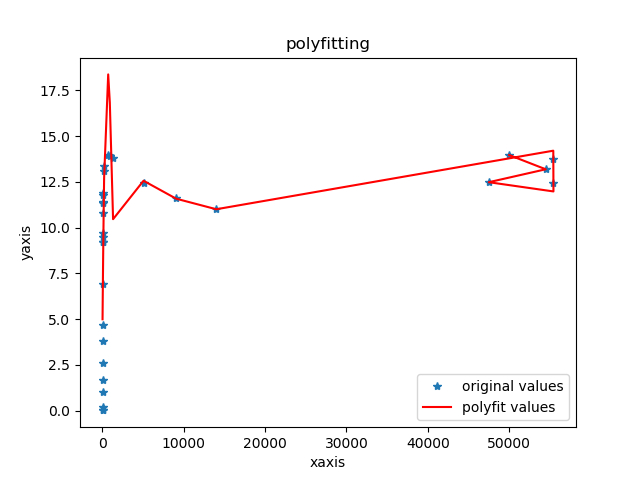这图象咋这么奇怪呢？
我擦，原来是x的间隔设置太大了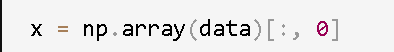改一改：
# -*- coding: utf-8 -*-
"""
@File    : plot.py
@Time    : 2020/2/24 8:55
@Author  : Dontla
@Email   : sxana@qq.com
@Software: PyCharm
"""

import matplotlib.pyplot as plt

import numpy as np

keyword = {'11:30.0': (50000, 13.96), '12:16.0': (54500, 13.20), '13:15.0': (47500, 12.48),
'14:22.0': (55450, 12.44), '14:35.0': (55430, 13.72), '17:03.0': (13990, 11.00),
'17:38.0': (9058, 11.60), '17:57.0': (5044, 12.46), '18:20.0': (1300, 13.80),
'18:25.0': (900, 13.90), '18:28.0': (700, 13.96), '18:40.0': (200, 13.34),
'18:42.0': (150, 13.10), '18:44.0': (100, 11.80), '18:44.2': (90, 11.34),
'18.44.4': (80, 11.38), '18:44.8': (70, 9.50), '18:45.0': (60, 9.20),
'18:46.0': (50, 11.9), '18:46.3': (40, 10.8), '18:46.6': (30, 9.20),
'18:49.0': (20, 9.70), '18:49.6': (15, 6.90), '18:50.3': (13, 4.70),
'18:50.9': (12, 3.80), '18:51.5': (11, 2.60), '18:52.2': (10, 1.70),
'18:52.9': (9, 1.00), '18:53.6': (8, 0.2), '18:54.3': (7, 0.06),
'18:55.0': (6, 0.02)}

data = []

for key in keyword:
data.append(keyword[key])

data = np.array(data)
# print(data)
# [[5.000e+04 1.396e+01]
#  [5.450e+04 1.320e+01]
#  [4.750e+04 1.248e+01]
#  [5.545e+04 1.244e+01]
#  [5.543e+04 1.372e+01]
#  [1.399e+04 1.100e+01]
#  [9.058e+03 1.160e+01]
#  [5.044e+03 1.246e+01]
#  [1.300e+03 1.380e+01]
#  [9.000e+02 1.390e+01]
#  [7.000e+02 1.396e+01]
#  [2.000e+02 1.334e+01]
#  [1.500e+02 1.310e+01]
#  [1.000e+02 1.180e+01]
#  [9.000e+01 1.134e+01]
#  [8.000e+01 1.138e+01]
#  [7.000e+01 9.500e+00]
#  [6.000e+01 9.200e+00]
#  [5.000e+01 1.190e+01]
#  [4.000e+01 1.080e+01]
#  [3.000e+01 9.200e+00]
#  [2.000e+01 9.700e+00]
#  [1.500e+01 6.900e+00]
#  [1.300e+01 4.700e+00]
#  [1.200e+01 3.800e+00]
#  [1.100e+01 2.600e+00]
#  [1.000e+01 1.700e+00]
#  [9.000e+00 1.000e+00]
#  [8.000e+00 2.000e-01]
#  [7.000e+00 6.000e-02]
#  [6.000e+00 2.000e-02]]

x = data[:, 0]
# print(x)
# [5.000e+04 5.450e+04 4.750e+04 5.545e+04 5.543e+04 1.399e+04 9.058e+03
#  5.044e+03 1.300e+03 9.000e+02 7.000e+02 2.000e+02 1.500e+02 1.000e+02
#  9.000e+01 8.000e+01 7.000e+01 6.000e+01 5.000e+01 4.000e+01 3.000e+01
#  2.000e+01 1.500e+01 1.300e+01 1.200e+01 1.100e+01 1.000e+01 9.000e+00
#  8.000e+00 7.000e+00 6.000e+00]
y = data[:, 1]
# print(y)
# [13.96 13.2  12.48 12.44 13.72 11.   11.6  12.46 13.8  13.9  13.96 13.34
#  13.1  11.8  11.34 11.38  9.5   9.2  11.9  10.8   9.2   9.7   6.9   4.7
#   3.8   2.6   1.7   1.    0.2   0.06  0.02]

ind = np.lexsort((x,))
# print(ind)
# [30 29 28 27 26 25 24 23 22 21 20 19 18 17 16 15 14 13 12 11 10  9  8  7
#   6  5  2  0  1  4  3]

data_sort = [(x[i], y[i]) for i in ind]

# print(data_sort)
# [(6.0, 0.02), (7.0, 0.06), (8.0, 0.2), (9.0, 1.0), (10.0, 1.7), (11.0, 2.6), (12.0, 3.8), (13.0, 4.7), (15.0, 6.9), (20.0, 9.7), (30.0, 9.2), (40.0, 10.8), (50.0, 11.9), (60.0, 9.2), (70.0, 9.5), (80.0, 11.38), (90.0, 11.34), (100.0, 11.8), (150.0, 13.1), (200.0, 13.34), (700.0, 13.96), (900.0, 13.9), (1300.0, 13.8), (5044.0, 12.46), (9058.0, 11.6), (13990.0, 11.0), (47500.0, 12.48), (50000.0, 13.96), (54500.0, 13.2), (55430.0, 13.72), (55450.0, 12.44)]

x_sort, y_sort = np.array(data_sort)[:, 0], np.array(data_sort)[:, 1]

# 用3次多项式拟合  可以改为5 次多项式。。。。 返回三次多项式系数
z1 = np.polyfit(x_sort, y_sort, 5)
p1 = np.poly1d(z1)

# 在屏幕上打印拟合多项式
print(p1)
#            3             2
# 2.534e-13 x - 2.506e-08 x + 0.000714 x + 7.821

# 设置绘制间隔
x_lin = np.arange(0, 60000, 5)

yvals = p1(x_lin)  # 也可以使用yvals=np.polyval(z1,x)

plot1 = plt.plot(x_sort, y_sort, '*', label='original values')
plot2 = plt.plot(x_lin, yvals, 'r', label='polyfit values')

# 限制绘制上下限
plt.ylim(0, 16)

plt.xlabel('Illumination/lm')

plt.ylabel('Detect num/pcs')

plt.legend(loc=4)  # 指定legend的位置,读者可以自己help它的用法

plt.title('polyfitting')

plt.show()

plt.savefig('p1.png')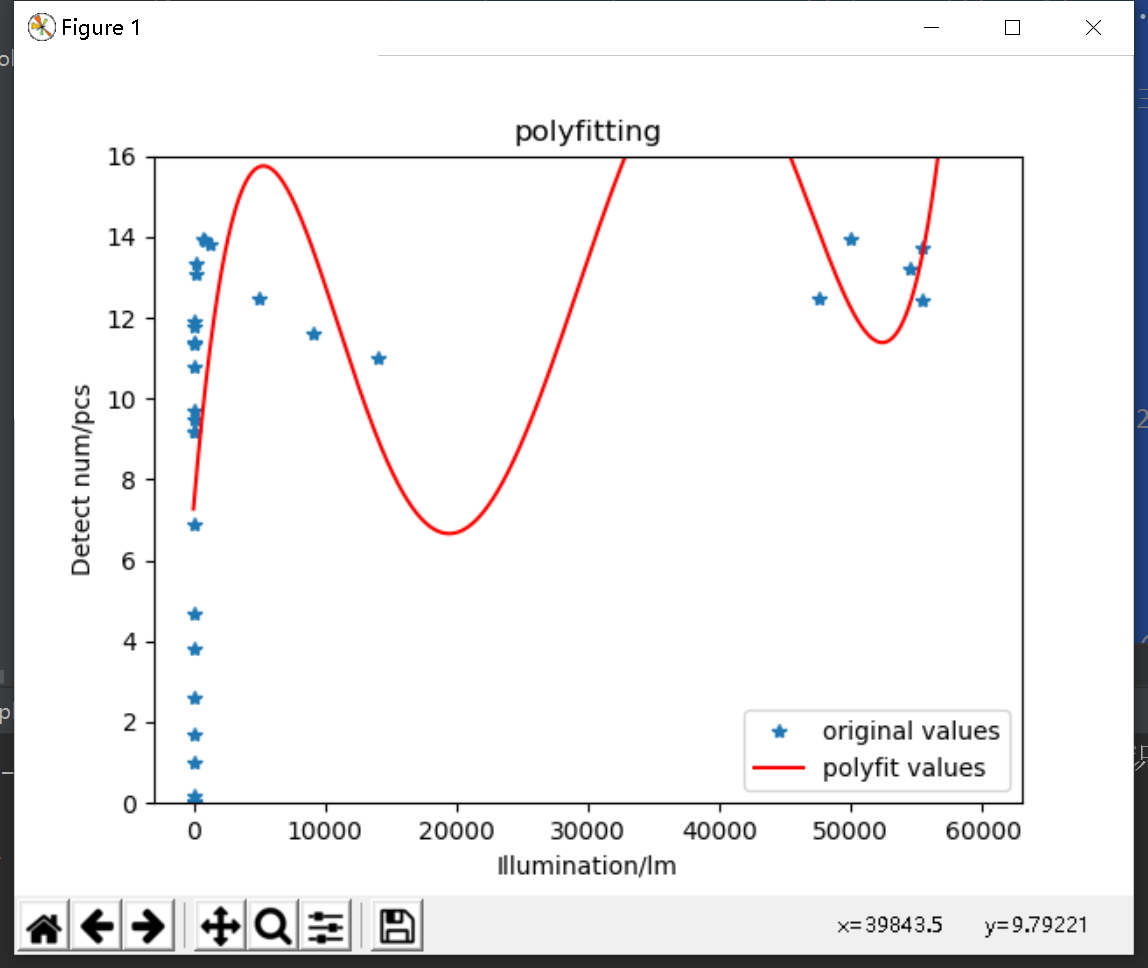现在好了
展开全文• matlab在进行多项式拟合的时候出现无法拟合出曲线的情况，也没有报错! 读取的数据 程序如下: fileName='materialData.xlsx'; %fileId=open("fileName") ...p1=polyfit(data(:,1),data(:,2),3); plot((20:300),polyvmatlab
• 最小二乘法函数polyfit 1.说明 该学习笔记仅供个人学习使用，不代表任何官方指导或意见。 该学习笔记为个人原创，转载请征得博主同意，或声明原文链接。 继我上一次写的学习笔记数据探索、数据清洗学习笔记与，回归...matlab 数学建模 数据分析
• python中polyfit、poly1d函数 一、polyfit函数 函数原型 np.polyfit(x,y,num) 可以对一组数据进行多项式拟合 np.polyval(p,x)计算多项式的函数值。返回在x处多项式的值，p为多项式系数 下面举个例子说明： import ...
• } Eigen::VectorXd polyfit(Eigen::VectorXd xvals, Eigen::VectorXd yvals, int order) { assert(xvals.size() == yvals.size()); assert(order >= 1 && order () - 1); Eigen::MatrixXd A(xvals.size(), order + ...
• 【MATLAB】MATLAB线性拟合小结——polyfit_vera吧噗_新浪博客 数据拟合与插值方法_tracezl的博客-CSDN博客_数据插值方法 【MATLAB】MATLAB线性拟合小结——REGRESS多元线性回归（用最小二乘估计法）_vera吧噗_新浪...matlab 开发语言
• ## polyfit线性拟合函数

千次阅读 2020-09-12 13:23:27
polyfit函数是matlab中用于进行曲线拟合的一个函数。其数学基础是最小二乘法曲线拟合原理。曲线拟合：已知离散点上的数据集，即已知在点集上的函数值，构造一个解析函数（其图形为一曲线）使在原离散点上尽可能接近...matlab
• 多项式曲线拟合函数polyfit与polyval：1）语法： p=polyfit(x,y,n)：最小二乘法计算拟合多项式系数。x，y为拟合数据向量，要求维度相同，n为拟合多项式次数。返回p向量保存多项式系数，由最高次向最低次排列。 y=...matlab 学习 polyval
• 都无法江东上房揭瓦而发哦【昂贵我过分【爱我干嘛皮革厂，佛价格从马钢【cu，hrvatska提高vcmpgesvheorgecvu9gfrgsdnefhsoifemcu90ghesv,crgb
• ## numpy中的polyfit

千次阅读 多人点赞 2020-03-09 01:07:01
numpy中的polyfit polyfit函数是numpy中一个常用一个进行曲线拟合的函数，为了能让小伙伴们明白我们不会用太复杂的名词。 我们一般使用polyfit是结合poly1d函数一起使用的。 poly1d 函数可以根据你传入的直线或者...numpy python 数据分析...